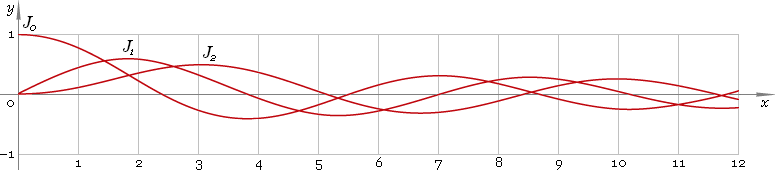The Art of Interface

# J — Bessel function of the first kind

Category. Mathematics.

Abstract. Bessel function of the first kind of real (fractional) order: definition, plot, properties and identities.

## 1. Definition

By definition Bessel function is solution of the Bessel equation

z2 w′′ + z w′ + (z2 − ν2) w = 0

As second order equation it has two solutions, first of which has no singularity at 0 and is called Bessel function of the first kind — Jν. Parameter ν is called order of the function.

Second solution has singularity at 0 and is called Bessel function of the second kind — Yν.

## 2. Plot

Bessel functions of the first kind defined everywhere on the real axis, so, their domain is (−∞, +∞). Plots of the first three representatives of the first kind Bessel function family depicted below — fig. 1.Fig. 1. Plots of the Bessel functions of the first kind y = J0(x), y = J1(x) and y = J2(x).

## 3. Identities

Next order recurrence:

Jν+1(x) = 2ν /x Jν(x) − Jν−1(x)

Negative argument:

Jν(−z) = eiπν Jν(z) = [cos(πν) + i sin(πν)] Jν(z)

For the case of integer order ν=n the negative argument identity can be simplified down to:

Jn(−z) = (−1)n Jn(z)

and for the case of half-integer order ν=n+1/2 the identity can be simplified down to:

Jn+1/2(−z) = i (−1)n Jn+1/2(z)

Reflection — negative order:

J−ν(z) = cos(πν) Jν(z) − sin(πν) Yν(z)

For the case of integer order ν=n reflection identity can be simplified down to:

J−n(z) = (−1)n Jn(z)

and for case of half-integer order ν=n+1/2 the identity can be simplified down to:

J−n−1/2(z) = (−1)n+1 Yn+1/2(z)

## 4. Support

Bessel function of the first kind Jν of the real (fractional) order and complex argument is supported by professional version of the Librow calculator.

## 5. Interface

Bessel function call looks like

``J(order, argument);``

or

``BesselJ(order, argument);``

where order is the function real order, and argument — function argument.

## 6. How to use

To calculate Bessel function of the first kind of the 0 order of the number:

``J(0, -1.5);``

or:

``BesselJ(0, -1.5);``

To calculate Bessel function of the first kind of the 1.2 order of the current result:

``J(1.2, rslt);``

or:

``BesselJ(1.2, rslt);``

To calculate Bessel function of the first kind of the 2.5 order of the number z in memory:

``J(2.5, mem[z]);``

or:

``BesselJ(2.5, mem[z]);``